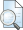# Hubungan Minat Belajar Dengan Hasil Belajar Matematika Penelitian Korelatif pada Siswa Kelas VIIIC Semester Ganjil SMP Negeri 3 Sawit Tahun Ajaran 2016/2017)

Istiqomah Fajriani, Nurul and , Idris Harta, M.A., Ph.D (2017) Hubungan Minat Belajar Dengan Hasil Belajar Matematika Penelitian Korelatif pada Siswa Kelas VIIIC Semester Ganjil SMP Negeri 3 Sawit Tahun Ajaran 2016/2017). Skripsi thesis, Universitas Muhammadiyah Surakarta.PDF (NASKAH PUBLIKASI) NASKAH PUBLIKASI r.pdf Download (739kB)PDF (HALAMAN DEPAN) HALAMAN DEPAN R.pdf Download (883kB)PDF (BAB I) BAB I.pdf Download (84kB)PDF (BAB II) BAB II.pdf Restricted to Repository staff only Download (164kB) | Request a copyPDF (BAB III) BAB III.pdf Restricted to Repository staff only Download (396kB) | Request a copyPDF (BAB IV) BAB IV.pdf Restricted to Repository staff only Download (213kB) | Request a copyPDF (BAB V) BAB V.pdf Restricted to Repository staff only Download (89kB) | Request a copyPDF (DAFTAR PUSTAKA) DAFTAR PUSTAKA VIKS.pdf Download (210kB)PDF (LAMPIRAN) LAMPIRAN OK.pdf Download (2MB)PDF (PERNYATAAN PUBLIKASI) PERNYATAAN PUBLIKASI.pdf Restricted to Repository staff only Download (308kB) | Request a copy

## Abstract

Interest has a very important role in the learning process because interest becomes one of the decisive factors in the success or failure of the goal of the teacher to be achieved. Students who are not interested in learning materials will show less sympathetic attitude, lazy and not enthusiastic follow the learning process. This research is a type of quantitative-correlative research conducted in the State Junior High School 3 Sawit Year 2016/2017 Lesson. Methods of data 2 collection using questionnaires for learning interest variables and documentation for mathematics learning outcome variables. Data analysis techniques use simple linear regression analysis. The result of research that simple regression result between student's learning interest and mathematics learning result of VIIIC student of SMP N 3 SAWIT of Lesson Year 2016/2017 obtained regression equation Y = 16.465 + 0.808 X. Interpretation of the result that the constant value of 16.465, meaning that if not variable interest in learning then the result of learning mathematics is constant 16,465. Then if there is a learning interest variable that increases one point then can give a positive contribution to mathematics learning result of regression coefficient equal to 0,808 with error rate in predict equal to 0,137 or 13,7%. The value of regression coefficient significance test of 5.913 and significance of 0.000. The value of simple correlation coefficient of 0.723 while the value (rtabel) of 0.339 so that the value of rxy (0.723)>rtabel (0.339), and the value of determination coefficient of 0.522 or 52.2%, it shows that the relationship of learning interest with the results of learning mathematics of 52,2% or in other words free variable able to explain dependent variable equal to 52,2% while the rest is 47,8% explained by other variable not included in research. So concluded there is a relationship between interest in learning with mathematics learning outcomes

Item Type: Karya ilmiah (Skripsi) Interest, Learning Outcomes, Mathematics L Education > LB Theory and practice of education > LB1603 Secondary Education. High schoolsQ Science > QA Mathematics Fakultas Keguruan dan Ilmu Pendidikan > Pendidikan Matematika NURUL ISTIQOMAH FAJRIANI 11 Aug 2017 03:33 11 Aug 2017 03:33 http://eprints.ums.ac.id/id/eprint/55136View Item